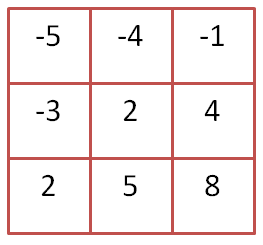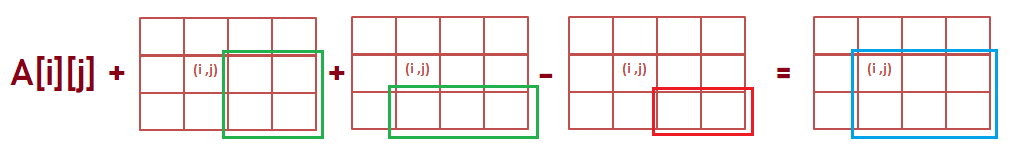# Kingdom War

## Introduction

Let’s discuss the problem statement first for better understanding.
So without any further ado, let’s begin.

## Problem Statement

Two kingdoms, Kingdom X and Kingdom Y are at war right now. As a war specialist of kingdom X, we have scouted the area of kingdom Y and plotted it in the form of a 2d grid. The kingdom grid is an NxM grid with each cell denoting a village. The cell value represents the strength of each village.

The cell values can be positive or negative. Positive value denotes the strength of the village. The negative value indicates the number of our warriors that have been held hostages.

The filling of the matrix has the following two constraints:

• The strength of a village on a row larger than 1 is stronger than or equal to the strength of the village, which is exactly above it.
• The strength of any village on a column larger than 1 is stronger than or equal to the strength of the village, which is exactly to its left.

Our task is to find out the largest sum of strength that we can erase by bombing one submatrix in the whole grid.

Example: Consider the matrix A as given below:Explanation: Output for the above grid will be 19. We can bomb the submatrix from (1,1) to (2,2). Power will be 2+4+5+8 = 19.

## Solution and Explanation

After reading the question, it is pretty clear that our task is to find a submatrix in the given matrix whose sum is the largest that can be erased by bombing.

Based on the above two constraints, we can assume that the largest submatrix sum may start from any cell in the grid but end on the bottom-right cell (N, M).

Therefore, we can use dynamic programming to find the sum of submatrices starting from the bottom-right cell (N,M) going up and left. Thus find the maximum answer from DP[i][j] for each (i,j),  where dp[i][j] stores the matrix sum from cell (i,j) to cell (n-1, m-1)

Where DP[i][j] = DP[i+1][j] + DP[i][j+1] - DP[i+1][j+1]

How does the above approach work?

For some cell (i, j) the matrix sum from (i, j) to (n-1, m-1)  will be nothing but

sum of matrix from (i+1, j)  to (n-1, m-1) + sum of matrix from (i, j+1)  to (n-1, m-1) + A[i][j].

But if we notice carefully we have added the matrix sum from (i+1, j+1) to (n-1, j-1) twice. Hence we need to subtract it once.### Implementation

Output

The output generated on running the above code is as follows:

### Time Complexity

In the code mentioned above, we traverse the whole input matrix once to find the various sub-matrices and make corresponding comparisons. Thus time complexity is,

T(n) = O(n*m)

where n is the number of rows and m is the number of columns.

### Space  Complexity

In the code mentioned above, we create a 2D dp table to store the matrix sum from cell (i,j) to cell (n-1, m-1).

Space Complexity = O(n*m)

where n is the number of rows and m is the number of columns.

## Optimized Approach

• Create a 1d dynamic table (dp) that will hold the sum of the row values for us.
• Now, Start iterating from the bottom-most row and work our way up. The size of our dynamic table will be the same as the number of columns in the input matrix.
• Create two temporary variables - let’s say temp and curr_maxsum
• Take another variable, say max_sum to store the final answer.
• Initialize temp with A, which is the first value of our input matrix.

Why do we do so, you may ask?

After reading the two constraints for the matrix filling given in the question, it is pretty clear that the smallest value of the matrix is at the first cell (0,0), and the largest value is at the last cell (N-1, M-1). So as the program execution starts and certain comparisons have been made, the maximum value of the grid is stored in temp.

• Now the second temporary variable is curr_maxsum. This variable is used to store the maximum submatrix sum. We compare the values of curr_maxsum (current maximum sum value) and max_sum (overall maximum sum value) and store the maximum of the two in max_sum.
• Finally return max_sum.

### Implementation

Output

The output generated on running the above code is as follows:

One may ask, how did we get 19 as the largest sum? The explanation is simple. Our task was to find the submatrix with the maximum sum starting from some cell but ending at the last cell. Thus the solution submatrix in our case begins from (1,1) up to (2,2), and the sum of the elements of this submatrix is 2+4+5+8 = 19.

### Time Complexity

In the code mentioned above, we traverse the whole input matrix once to find the various sub-matrices and make corresponding comparisons. Thus time complexity is,

T(n) = O(n*m)

where n is the number of rows and m is the number of columns.

### Space  Complexity

In the code mentioned above, we create a 1d table to store the various rows of our input matrix. Thus,

Space Complexity = O(m)

where m is the number of columns.

Q1. Is there any alternate solution to the Kingdom War problem?

Ans. Yes, there are multiple ways of solving the Kingdom War problem. It can be solved using recursion or iteration, but their complexities give cubic solutions. One of the most efficient solutions is by using Kadane’s Algorithm. If you wish to know more about the same, please read this blog: Kadane's Algorithm

## Key Takeaways

To summarize this article, we have discussed the Kingdom War problem thoroughly. We first read and analyzed the given problem statement. After understanding the problem, we came up with an optimized solution that involved Dynamic Programming concepts, followed by some frequently asked questions.
Want to ace the coding rounds of big tech companies? Try our Attempt Unlimited Online Mock Test Series to start your preparation.